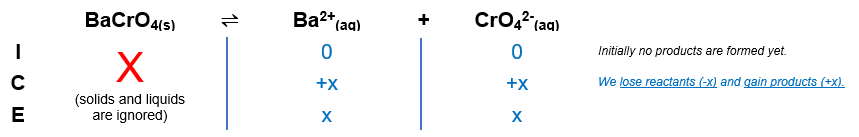# Problem: Use the given molar solubilities in pure water to calculate Ksp for each compound. a. BaCrO4; molar solubility = 1.08 x 10-5 M b. Ag2SO3; molar solubility = 1.55 x 10-5 M c. Pd(SCN)2; molar solubility = 2.22 x 10-8 M

###### FREE Expert Solution

We're being asked to calculate the Ksp for each compound

a. BaCrO4; molar solubility = 1.08 x 10-5

The dissociation of BaCrO4 in water is as follows:
BaCrO4(s) → Ba2+(aq) + CrO42(aq)

We can construct an ICE table for the dissociation of BaCrO4

Remember that solids are ignored in the ICE table and Ksp expression.The Ksp expression for BaCrO4 is:

87% (95 ratings)###### Problem Details

Use the given molar solubilities in pure water to calculate Ksp for each compound.

a. BaCrO4; molar solubility = 1.08 x 10-5

b. Ag2SO3; molar solubility = 1.55 x 10-5 M

c. Pd(SCN)2; molar solubility = 2.22 x 10-8 M